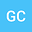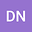Mathematical aspects of fluid-multiferroic solid interaction problems
••• David Natroshvili
I. Javakhishvili Tbilisi State University

Author ProfileDavid Natroshvili
Georgian Technical University
Author Profile## Abstract

In the paper, we consider a three-dimensional model of fluid-solid interaction when a thermo-electro-magneto-elastic body occupying a bounded region $$\Omega^{+}$$ is embedded in an inviscid fluid occupying an unbounded domain $$\Omega^{-}=\mathbb{R}^{3}\setminus\overline{\Omega^{+}}$$. In this case, we have a six-dimensional thermo-electro-magneto-elastic field (the displacement vector with three components, electric potential, magnetic potential, and temperature distribution function) in the domain $$\Omega^{+}$$, while we have a scalar acoustic pressure field in the unbounded domain $$\Omega^{-}$$. The physical kinematic and dynamic relations are described mathematically by appropriate boundary and transmission conditions. With the help of the potential method and theory of pseudodifferential equations, we prove the uniqueness and existence theorems for the corresponding boundary-transmission problems in appropriate Sobolev-Slobodetskii and Hölder continuous function spaces.

10 Mar 2020Submitted to Mathematical Methods in the Applied Sciences
20 Mar 2020Assigned to Editor
20 Mar 2020Submission Checks Completed
27 Mar 2020Reviewer(s) Assigned
17 Nov 2020Review(s) Completed, Editorial Evaluation Pending
17 Nov 2020Editorial Decision: Accept